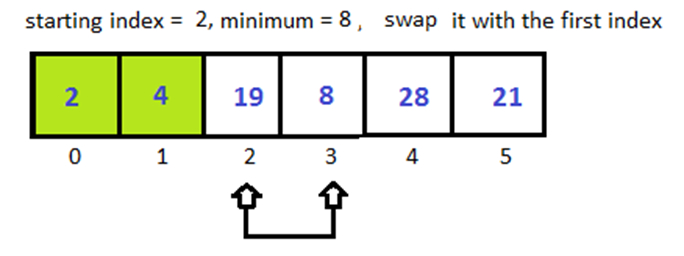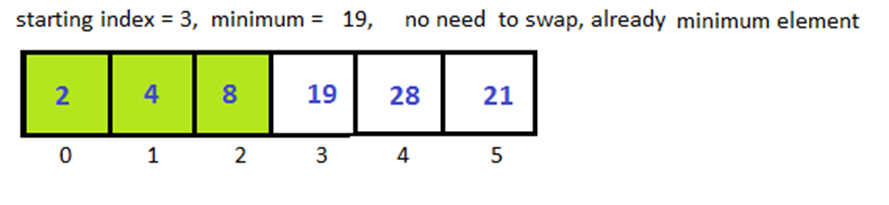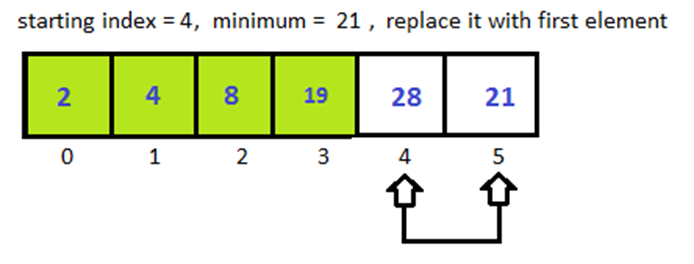# Python Selection Sort

Here you’ll learn about python selection sort algorithm with program example.

Selection sort is one of the easiest sorting algorithm out there. In Selection sort, to sort an unsorted array, all we have to do is find the minimum in the array and swap it with the first element in the unsorted array. After each swapping increase the starting index of the array by one.

This is for sorting in ascending order. If we want to sort it into descending order find the maximum instead of minimum and replace with the first element in unsorted array.

## Python Selection Sort

### Example

We have an unsorted array-

[4,8,19,2,28,21]

Step 1:Step 2:Step 3:Step 4:Step 5:Step 6:### Algorithm

If we have an array of n elements.

Step 1:- set MIN = 0

Step 2:- find minimum element in array

If exists then swap with element at MIN

Step 3:- increase MIN by 1

Step 4:- go to Step 2 until  array is sorted (for n-1 times)

### Time Complexity

• Best Case = O(n)2
• Average Case = O(n)2
• Worst Case = O(n)2

Note: for larger arrays we never use selection sort.

### Python Selection Sort Program

Below is the implementation of selection sort in python.

Output

2 4 8 19 21 28

Comment below if you have any queries related to above python selection sort tutorial.

### 2 thoughts on “Python Selection Sort”

1.how will know about no of guesses or pass

2.i need the program for sorting like,
Step 1: Import the randint definition of the random library of python. Check this page if you want some help.

Step 2: Take the elements of the list_1 as input.

Step 3: randomly choose two indexes i and j within the range of the size of list_1.

Step 4: Swap the elements present at the indexes i and j. After doing this, check whether the list_1 is sorted or not.

Step 5: Repeat step 3 and 4 until the array is not sorted.

can u do this in python?